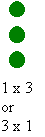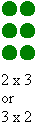# Mathematician of the Week – Spring 2 – Week 1 & 2

We have got not one but two Mathematicians of the Week this week!We have started to look at a new topic of multiplication and these two superstars are working so hard to apply their knowledge of multiplication when answering reasoning and problem solving questions! Excellent work!

# Mathematician of the Week

Our mathematician of the week is a superstar! You have tried so hard to answer your length word problems by yourself, you recognised which operation to complete and you could show clear working out. Fantastic! 🙂# Mathematician of the Week

Well done to our Mathematician of the Week. He was so surprised when he heard that he was the winner this week.You have impressed all of the adults in Pearl this week with your attitude to your maths work, the ability to show your working out and you can explain how you found your answers to explain how multiplication and division are linked. Well done 🙂

# Arrays, arrays, arrays

In maths, we have been learning how to can use arrays to help us to complete multiplication questions and then we had to answer some really tricky word problems.

### We can using arrays to explore numbers

Arrays are useful models for multiplication which can be used in a variety of ways, ranging from highly structured lessons to games and open investigations. An array is formed by arranging a set of objects into rows and columns. Each column must contain the same number of objects as the other columns, and each row must have the same number as the other rows.

The following array, consisting of four columns and three rows, could be used to represent the number sentence 3 x 4 = 12.### We can build number facts

Arrays can be used for building multiplication facts in a meaningful way. Before drilling and memorising tables, children must understand how these facts are derived. For example, by progressively adding another column of three objects, children can build the three-times tables for themselves. This representation not only assists in understanding the process, but provides a visual image for children to draw upon as they begin to use and memorise the basic number facts.How to continue the learning at home:

• Practise counting with your child in steps of 2, 3, 5 and 10 so that they can become fluent.

# Mathematician of the Week

Well done to our first Mathematician of the Week of 2017.We have been learning about how to use arrays in maths this week to help us with our multiplication. You were so quick at answering the multiplication word problems and showing really clear working out with arrays to help you to find the answer. Well done! 🙂

Today we started to learn about multiplication and how we could use repeated addition to help us to find our answers. We are really good at counting in steps of 2, 5 and 10 so we decided that we would create multiplication number sentences using facts that we already knew.

We have learnt that the symbol x can also mean ‘lots of’.

2 x 5 is the same as 2 lots of 5 which is the same as 5 + 5

We used counters and coins to help us to record our working out.

How you can continue the learning at home:

• Practise counting in steps of 2, 5 and 10 so that the children are really familiar with it.
• Learn the times tables for 2, 5 and 10 and be able to recite them off by heart.
• Write some simple multiplication number sentences for your child to answer and then bring them into school to show Miss Varley what you have been learning about at home.